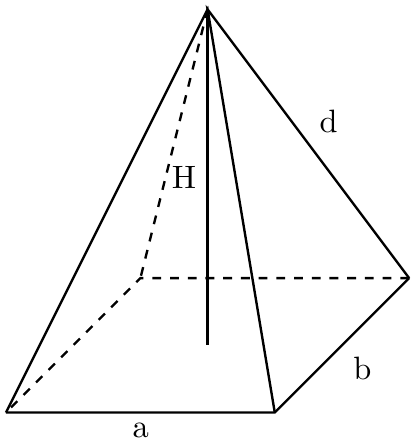# Rectangular Pyramid Volume Calculator

Created by Purnima Singh, PhD
Last updated: Feb 02, 2023

The rectangular pyramid volume calculator can help you find the volume and surface area of a pyramid with a rectangular base.

• What a rectangular pyramid is; and
• How to find the volume of a rectangular-based pyramid?

You will also find an example of using the rectangular pyramid volume calculator.

## How to find the volume of a rectangular pyramid - formulas

A rectangular pyramid is a polyhedron (three-dimensional shape) with a rectangular base and triangular lateral faces (see figure 1). Some famous examples of rectangular pyramids are the Egyptian pyramids and the Louvre pyramid.

To calculate the volume or capacity of a rectangular pyramid, we will use the formula:

$\quad V = \frac{a b H}{3}$

where:

• $a$ - Length of the rectangular base;
• $b$ - width of the rectangular base; and
• $H$ - Height of the pyramid.

The formula for calculating the surface area of the pyramid is:

$A = ab + a \sqrt {\Big(\frac{b}{2}\Big)^2 + H^2} + b\sqrt {\Big(\frac{a}{2}\Big)^2 + H^2}$

## How to use the rectangular pyramid volume calculator?

Let us see how we can use the rectangular pyramid volume calculator to find the volume of a rectangular pyramid with length and width of base edges as 7 cm and 5 cm, respectively, and height as 10 cm.

1. Enter the dimensions of the base, i.e., base length = 7 cm and base width = 5 cm.

2. Type the height of the pyramid, i.e., 10 cm.

3. The calculator will display the total surface area (160.13 cm2) and volume of the rectangular based pyramid (116.67 cm3).

## Other pyramid calculators

We hope you enjoyed using our rectangular pyramid volume calculator. Make sure to check out our other tools that deal with the determination of various parameters of a pyramid.

## FAQ

### How do I get the volume of a rectangular pyramid?

To get the volume of a rectangular pyramid, follow the given instructions:

1. Multiply the length and width of the rectangular base to get its area.

2. Now multiply the base area with the height of the pyramid.

3. Divide the result from step 2 by three, and you will get the volume of a rectangular pyramid.

### How many faces does a rectangular pyramid have?

A rectangular pyramid has five faces and eight edges. Out of these five faces, the base face is rectangular and the other four faces are triangular-shaped.

### How many vertices are there in a rectangular pyramid?

There are five vertices in a rectangular pyramid. One vertex is located above the rectangular base of the pyramid. The other four vertices lie at the four corners of the base.

Purnima Singh, PhDBase length (a)
in
Base width (b)
in
Pyramid height (H)
in
Pyramid parameters
Total surface area (A)
in²
Volume (V)
cu in
People also viewed…

### Arithmetic sequence

The arithmetic sequence calculator finds the nᵗʰ term and the sum of a sequence with a common difference.

### Christmas tree

Welcome to the Christmas tree calculator, where you will find out how to decorate your Christmas tree in the best way. Take a look at the perfect Christmas tree formula prepared by math professors and improved by physicists. Plan in advance how many lights and decorations you'll need!